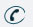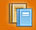781-366-0662

Subject

## Major Areas of Study

Functions, Graphs, and Limits : Students should be able to work with functions represented in a variety of ways: graphical, numerical, analytical, or verbal. They should understand the connections among there representations.

Topics Include : Functions, Limits, and Continuity, Families of Curves

Derivatives : Students should understand the meaning of the derivative in terms of a rate of change and local linear approximation and should be able to use derivatives to solve a variety of problems.

Topics Include : Differentiability, Derivatives From Graphs/Charts, Tangent Line Problems, Power, Product and Quotient Rules, Trig. Derivatives, Second Derivatives, Chair Rule, Linear Approximations, Implicit Differentiation, Composite, Inverse, Exponential and Logarithmic Functions and Derivatives, Inverse Trigonometric Derivatives, Graphing and Continuity, Extreme Value Theorem, Mean Value Theorem, Candidates’ Test, Relative and Absolute Maxima and Minima, M2I2ACIDS, Related Rates, Velocity and Acceleration, Estimating Areas with Finite Sums, Left, Right, Upper, and Lower Trapezoidal Methods.

Integrals : Students should understand the meaning of the definite integral both as a limit of Riemann sums and as the net accumulation of change and should be able to use integrals to solve a variety of problems. In addition, they should understand the relationship between the derivative and the definite integral as expressed in both parts of the Fundamental Theorem of Calculus.

Topics Include : Integration, Initial Value, Definite Integrals, Fundamental Theorem, Separation of Variables, Slope Fields, Integration of Trigonometric Functions, Areas Between Curves, Integration by Parts and Substitution, Volume of Discs/Washers, and Shells.Subject Classes